# Transversality

(diff) ← Older revision | Latest revision (diff) | Newer revision → (diff)

The general name for certain ideas of general position (cf. also Transversal mapping); a concept in linear algebra, differential and geometric topology.

a) Two vector subspacesof a finite-dimensional vector spaceare transversal to one another ifandgenerate, that is, ifb) In the differentiable situation, two submanifolds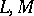of a manifoldare transversal at a pointif the tangent spaces,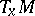at this point generate. Geometrically (for submanifolds in the narrow sense of the word and without boundary) this means that it is possible to introduce local coordinatesintoin some neighbourhoodof, in terms of which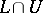and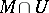are represented as transversal vector subspaces of.

A mappingis transversal to a submanifoldat a point(cf. Transversal mapping) if the image ofunder the differential ofis transversal toin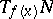. Two mappings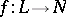and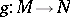are transversal to each other at a point, where, if the images of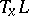and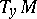generate. The latter two definitions can also be rephrased geometrically . One says thatis transversal to, andto(in old terminology:is-regular along), andto, if the corresponding transversality holds at all points for which it is possible to talk about it. These concepts easily reduce to one another. E.g. the transversality ofandis equivalent to the transversality of the identity imbeddings ofandin. In common use are the notations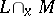,, etc.

For transversality of manifolds with boundary it is sometimes useful to require certain extra conditions to hold (see ). Transversality also carries over to the infinite-dimensional case (see , ).

In all these situations the role of transversality is connected with "genericity" and with the "good" properties of the intersection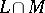, the pre-images, and analogous objects (which are deformed to the same "good" objects, if under the deformation of the original objects transversality is preserved) (see ).

c) In piecewise-linear and topological situations, transversality of submanifolds is defined similarly to the geometric definition in b). (Especially widespread is the piecewise-linear version for submanifolds of complementary dimension, cf. .) In general, one does not obtain a complete analogy with the properties of transversality in b) (see , ), therefore more restricted modifications of transversality have been proposed (see , ).

Finally, a category of manifolds is said to have the transversality property if any mapping in it can be approximated by a transversal mapping.

How to Cite This Entry:
Transversality. Encyclopedia of Mathematics. URL: http://encyclopediaofmath.org/index.php?title=Transversality&oldid=17887
This article was adapted from an original article by D.V. Anosov (originator), which appeared in Encyclopedia of Mathematics - ISBN 1402006098. See original article